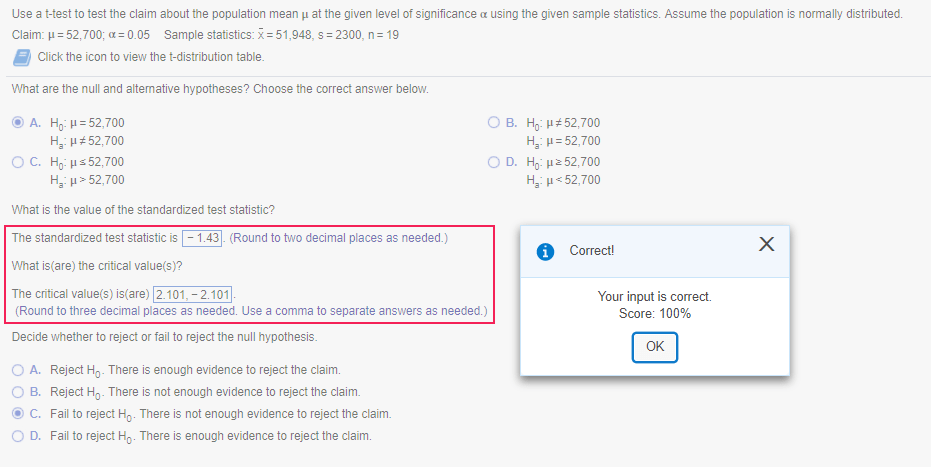# Rounding in MyStatLabI get frequent emails from students complaining that they had the right answer, but MyStatLab did not give them credit. Often, when I am reviewing their claim, I find that they did not enter the required number of decimal places. But sometimes, I find the student mistake was rounding prematurely in an intermediate step.

The general rule is to not round any intermediate values in a series of calculations and, instead, carry forward as many decimal places as possible. For example, if you need to first calculate the standard deviation for use in a later equation, keep as many decimal places as possible (no less than 6 is my recommendation) in your standard deviation value.

But when you enter your answers into MyStatLab (MSL), round to the required number of decimal places. When MSL says “two decimal places as required” or “one decimal place as needed,” that means exactly that many of decimal places are required.

In this example from 7.3, MSL asks for the “nearest thousandth as needed.”  That means you must enter exactly three numbers after the decimal.If you enter 2.85, you will be counted wrong. If you enter 2.8449, you will be counted wrong.  For example, see images below:Generally, MSL does allow a tolerance level around the exact answer, e.g. 4.2004 + or – 0.0001. And on some problems, MSL also includes alternate answers where “technology” gives a slightly different value than interpolating from a table, again within a tolerance range.

But when it comes to the number of decimal places, MSL is strict.

This may seem a bit picky, but in the real world, our bosses will not be happy if we do not follow instructions.

This site uses Akismet to reduce spam. Learn how your comment data is processed.